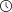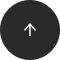- 10 minutes read

# SQL Statistical Analysis Part 1: Calculating Frequencies and Histograms

Database and Business Intelligence (BI) developers create huge numbers of reports on a daily basis, and data analyses are an integral part of them. If you wonder whether you can perform statistical analysis in SQL, the answer is ‘yes’. Read my article to learn how to do this!

Statistics are very useful as an initial stage of a more in-depth analysis, i.e. for data overview and data quality assessment. However, SQL statistical analysis possibilities are somewhat limited as there are not many statistical functions in SQL Server. . In addition, a good understanding of statistics is not very common among T-SQL practitioners. In SQL Server 2016, you can use R to calculate all kinds of statistical measures, but many SQL Server developers and database administrators do not program in R. And not every site has upgraded to SQL Server 2016.

This series will explain the basics of SQL statistical analysis. The code used is based on my real-life experiences. I deal with BI projects, especially with data mining, and I often need to create a lot of statistical queries in the initial stages of a project. During these projects, many times the only software I can rely on is an RDBMS.

### Optimizing SQL Statistical Queries

Optimizing statistical queries is different than optimizing transactional queries. In order to calculate the statistics, the query typically scans all the data. If the query is too slow, you can prepare a random sample of your data and scan that. However, if the queries blindly follow the formulas, they often perform multiple scans of the data. Optimizing such queries means minimizing the number of scans. To achieve this, you must develop an algorithm that uses additional mathematics to convert the formulas to equivalents that can be better optimized in SQL Server or any other RDBMS. You also need to understand SQL in depth. For example, you need a really good understanding of SQL’s window functions and calculations.

Besides explaining statistics and statistical queries, this series will also give you some ideas for optimizing statistical and non-statistical queries.

### Preparing Your Data for SQL Statistical Analysis

Before starting the analysis, you need to understand what you are analyzing. In statistics, you analyze cases using their variables. In RDBMS terminology, you can think of a case as a table row and a variable as a column in the same table. For most statistical analyses, you prepare a single table or view. Sometimes it is not so easy to exactly define your case. For example, if you’re performing a credit risk analysis, you might define a family as a case rather than a single customer.

When you prepare data for SQL statistical analysis, you have to transform the source data accordingly. For each case, you need to encapsulate all available information in the columns of the table you are going to analyze.

#### Continuous and Discrete Variables

Before starting a serious data overview, you must understand how data values are measured in your data set. You might need to check this with a subject matter expert and analyze the business system that is the source for your data. There are several ways to measure data values and different types of columns:

• Discrete variables can take a value only from a limited domain of possible values. Discrete values include categorical or nominal variables that have no natural order. Examples include states, status codes, and colors.
• Ranks can also take a value only from a discrete set of values. They have an order but do not permit any arithmetic. Examples include opinion ranks and binned (grouped, discretized) true numeric values.
• There are also some specific types of categorical variables. Single-valued variables or constants are not very interesting for analysis because they do not contribute any information. Two-valued or dichotomous variables have two values that are minimally needed for any analysis. Binary variables are specific dichotomous variables that take on only the values 0 and 1.
• Continuous variables can take any of an unlimited number of possible values; however, the domain itself can have a lower and/or upper boundary.
• Intervals have one or two boundaries, have an order, and allow some arithmetic-like subtraction (but may not always allow summation). Examples include dates, times, and temperatures.
• True numeric variables support all arithmetic. Examples include amounts and values.
• Monotonic variables are a specific type of continuous variables which increase monotonously without bound. If they are simply IDs, they might not be interesting. Still, they can be transformed (binned into categories) if the ever-growing ID contains time order information (lower IDs are older than higher IDs).

#### Data Used for SQL Statistical Analyses

For this and all upcoming articles, I am using the AdventureWorksDW2014 demo database. You can download a full backup of this database from Microsoft’s SQL Server sample site. I am running all of the code on SQL Server 2016 Developer Edition.

I prefer to use the AdventureWorks sample database for SQL Server 2014 instead of SQL Server 2016’s WideWorldImportersDW sample database. The WideWorldImporters database is very useful for demonstrating SQL Server 2016’s new features, but its data is lacking the correlations and associations needed for statistical analysis.

### Using Frequency Distribution in SQL to Understand Discrete Variables

In SQL, frequency distribution (usually presented in the form of a table) is used to get a quick overview of discrete variables. It can show the actual values as well as their:

• Absolute frequency
• Absolute percentage
• Cumulative frequency
• Cumulative percent

Plus, SQL frequency distribution displays a histogram of the values’ absolute percentage.

Below, I’ll show you several ways to compute frequency distribution in SQL, starting with one that is fairly inefficient.

#### Frequency Distribution in SQL without Using Window Functions

Calculating values’ absolute frequency and absolute percentage is a straightforward aggregation. However, calculating the cumulative frequency and cumulative percentage means calculating running totals. Before SQL Server 2012 added support for window aggregate functions, you had to use either correlated subqueries or non-equi self joins for this task. Neither method is very efficient.

Run the following code, which uses correlated subqueries, to analyze in SQL frequency distribution of the NumberCarsOwned variable from the dbo.vTargetMail view in the AdventureWorksDW2014 demo database.

```USE AdventureWorksDW2014;
GO
WITH freqCTE AS
(
SELECT v.NumberCarsOwned,
COUNT(v.NumberCarsOwned) AS AbsFreq,
CAST(ROUND(100. * (COUNT(v.NumberCarsOwned)) /
(SELECT COUNT(*) FROM vTargetMail), 0) AS INT) AS AbsPerc
FROM dbo.vTargetMail AS v
GROUP BY v.NumberCarsOwned
)
SELECT c1.NumberCarsOwned AS NCars,
c1.AbsFreq,
(SELECT SUM(c2.AbsFreq)
FROM freqCTE AS c2
WHERE c2.NumberCarsOwned &amp;lt;= c1.NumberCarsOwned) AS CumFreq,
c1.AbsPerc,
(SELECT SUM(c2.AbsPerc)
FROM freqCTE AS c2
WHERE c2.NumberCarsOwned &amp;lt;= c1.NumberCarsOwned) AS CumPerc,
CAST(REPLICATE('*',c1.AbsPerc) AS varchar(100)) AS Histogram
FROM freqCTE AS c1
ORDER BY c1.NumberCarsOwned;
```

This generates the following output:

```NCars  AbsFreq  CumFreq  AbsPerc  CumPerc  Histogram
-----  -------  -------  -------  -------  -----------------------------------
0         4238     4238       23       23  ***********************
1         4883     9121       26       49  **************************
2         6457    15578       35       84  ***********************************
3         1645    17223        9       93  *********
4         1261    18484        7      100  *******
```

#### Frequency Distribution in SQL Using Window Functions – Solution 1

When you perform a SQL statistical analysis, window aggregate functions prove useful. They provide a much better solution. As already noted, these functions are available in SQL Server versions 2012 and later.

If you look at the first part of the query, you’ll notice that the Common Table Expression query that calculates the absolute numbers is the same as in the previous query. However, the cumulative values – the running totals – are calculated with the help of window aggregate functions.

```WITH freqCTE AS
(
SELECT v.NumberCarsOwned,
COUNT(v.NumberCarsOwned) AS AbsFreq,
CAST(ROUND(100. * (COUNT(v.NumberCarsOwned)) /
(SELECT COUNT(*) FROM vTargetMail), 0) AS INT) AS AbsPerc
FROM dbo.vTargetMail AS v
GROUP BY v.NumberCarsOwned
)
SELECT NumberCarsOwned,
AbsFreq,
SUM(AbsFreq)
OVER(ORDER BY NumberCarsOwned
ROWS BETWEEN UNBOUNDED PRECEDING
AND CURRENT ROW) AS CumFreq,
AbsPerc,
SUM(AbsPerc)
OVER(ORDER BY NumberCarsOwned
ROWS BETWEEN UNBOUNDED PRECEDING
AND CURRENT ROW) AS CumPerc,
CAST(REPLICATE('*',AbsPerc) AS VARCHAR(50)) AS Histogram
FROM freqCTE
ORDER BY NumberCarsOwned;
```

The result of this query is the same as the result of the previous query.

#### Frequency Distribution in SQL Using Window Functions – Solution 2

I found another interesting solution using SQL’s window analytic functions. The CUME_DIST function calculates the cumulative distribution, or relative position, of a value in a group of values. For a row r, assuming ascending ordering, the CUME_DIST of r is the number of rows with values lower than or equal to the value of r, divided by the number of rows evaluated in the partition or query result set. The PERCENT_RANK function calculates the relative rank of a row within a group of rows. We can use PERCENT_RANK to evaluate the relative standing of a value within a query result set or partition.

The following SQL statistical analysis query calculates the row number once partitioned over the NumberCarsOwned column and the row number once over all the input set. It also calculates the percent rank and the cumulative distribution over the complete input set.

```SELECT NumberCarsOwned AS NCars,
ROW_NUMBER() OVER(PARTITION BY NumberCarsOwned
ORDER BY NumberCarsOwned, CustomerKey) AS Rn_AbsFreq,
ROW_NUMBER() OVER(
ORDER BY NumberCarsOwned, CustomerKey) AS Rn_CumFreq,
PERCENT_RANK()
OVER(ORDER BY NumberCarsOwned) AS Pr_AbsPerc,
CUME_DIST()
OVER(ORDER BY NumberCarsOwned, CustomerKey) AS Cd_CumPerc
FROM dbo.vTargetMail;
```

The partial output, showing only those rows pertinent to the explanation of the calculating frequencies algorithm, is:

```NCars  Rn_AbsFreq  Rn_CumFre  Pr_AbsPerc         Cd_CumPerc
-----  ----------  ---------  -----------------  --------------------
0               1          1                 0   5.4100843973166E-05
0               2          2                 0   0.000108201687946332
…               …          …                 …   …
0            4238       4238                 0   0.229279376758277
1               1       4239  0.22929178163718   0.229333477602251
…               …          …                 …   …
1            4883       9121  0.22929178163718   0.493453797879247
2               1       9122  0.493480495590543  0.49350789872322
…               …          …                 …   …
```

As you can see, the last row number partitioned by NumberCarsOwned in a category actually represents the absolute frequency of the values in that category. The last un-partitioned row number in a category represents the cumulative frequency up to and including the current category. For example, the absolute frequency for NumberCarsOwned = “0” is 4,238 and the cumulative frequency is 4,238; for NumberCarsOwned = “1”, the absolute frequency is 4,883 and the cumulative frequency is 9,121.

Next, consider the CUME_DIST function (the Cd_CumPerc column in the output). CUME_DIST in the last row in a category returns the cumulative percentage up to and including the category. If you subtract the PERCENT_RANK (the Pr_AbsPerc column in the output) for the last row in the category from the CUME_DIST of the last row in the same category, you get the absolute percentage for the category. For example, the absolute percentage for the category where NumberCarsOwned = “1” is more than 26 percent (0.493453797879247 – 0.22929178163718 = 0.264162016242067).

The following query calculates the frequencies distribution using observations from the results of the previous query.

```WITH freqCTE AS
(
SELECT NumberCarsOwned,
ROW_NUMBER() OVER(PARTITION BY NumberCarsOwned
ORDER BY NumberCarsOwned, CustomerKey) AS Rn_AbsFreq,
ROW_NUMBER() OVER(
ORDER BY NumberCarsOwned, CustomerKey) AS Rn_CumFreq,
ROUND(100 * PERCENT_RANK()
OVER(ORDER BY NumberCarsOwned), 0) AS Pr_AbsPerc,
ROUND(100 * CUME_DIST()
OVER(ORDER BY NumberCarsOwned, CustomerKey), 0) AS Cd_CumPerc
FROM dbo.vTargetMail
)
SELECT NumberCarsOwned AS NCars,
MAX(Rn_AbsFreq) AS AbsFreq,
MAX(Rn_CumFreq) AS CumFreq,
MAX(Cd_CumPerc) - MAX(Pr_Absperc) AS AbsPerc,
MAX(Cd_CumPerc) AS CumPerc,
CAST(REPLICATE('*',MAX(Cd_CumPerc) - MAX(Pr_Absperc)) AS varchar(100)) AS Histogram
FROM freqCTE
GROUP BY NumberCarsOwned
ORDER BY NumberCarsOwned;
```

Although the idea of this last query is very interesting, this query is not as efficient as the second one (using the window aggregate function). Therefore, the second solution is the recommended one.

### Conclusion

In this article, you learned how to compute SQL frequency distribution for discrete variables. You have also seen a solution that uses some creativity. In the following articles, you will get a grasp of other SQL statistical analysis methods. The next one will be dedicated to calculating basic statistical measures for continuous variables. You will also see how to write efficient queries that call for mathematical knowledge rather than creativity.